### Home > MC2 > Chapter 3 > Lesson 3.2.2 > Problem3-71

3-71.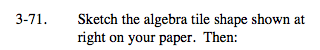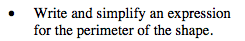The perimeter is the sum of all the side lengths that do not touch another tile.

Label and add all the side lengths together:
x + x + ( x − 1) + x + 1 + x + x
Simplify this expression.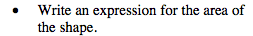The area of each tile is shown in the center of it.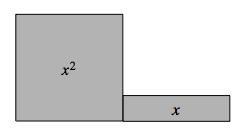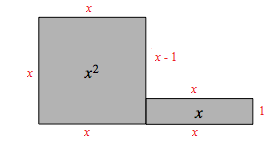Add the two areas together: x2 + x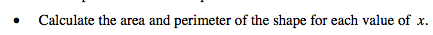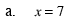Replace x with 7 in both equations.

Area = 72 + 7
Perimeter = 6(7)

Area = 56 sq. un.
Perimeter = 42 un.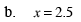See part (a).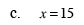See part (a).